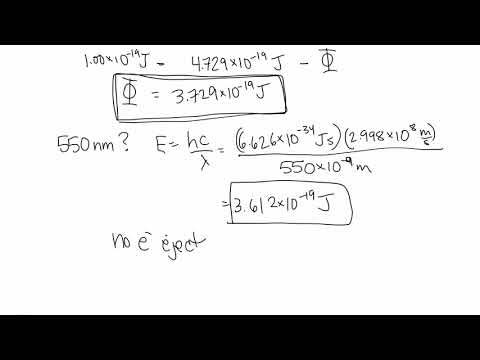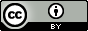9 minutes8

Electromagnetic radiation can be generated by exciting matter to higher energies, such as by heating it. The emitted light can be either continuous (incandescent sources like the sun) or discrete (from specific types of excited atoms). Continuous spectra often have distributions that can be approximated as blackbody radiation at some appropriate temperature. The line spectrum of hydrogen can be obtained by passing the light from an electrified tube of hydrogen gas through a prism.
Keywords: Blackbody radiation, Light, Photoelectric effect

## 8.1 Blackbody Radiation and the Ultraviolet Catastrophe

### Learning Objectives

By the end of this section, you should be able to:

• Explain the phenomenon of blackbody radiation

The last few decades of the nineteenth century witnessed intense research activity in commercializing newly discovered electric lighting. This required obtaining a better understanding of the distributions of light emitted from various sources being considered. Artificial lighting is usually designed to mimic natural sunlight within the limitations of the underlying technology. Such lighting consists of a range of broadly distributed frequencies that form a continuous spectrum. Figure 8.1 shows the wavelength distribution for sunlight. The most intense radiation is in the visible region, with the intensity dropping off rapidly for shorter wavelength ultraviolet (UV) light, and more slowly for longer wavelength infrared (IR) light.

Figure 8.1

The spectral distribution (light intensity vs. wavelength) of sunlight reaches the Earth's atmosphere as UV light, visible light, and IR light. The unabsorbed sunlight at the top of the atmosphere has a distribution that approximately matches the theoretical distribution of a blackbody at 5250 °C, represented by the blue curve. (credit: modification of work by American Society for Testing and Materials (ASTM) Terrestrial Reference Spectra for Photovoltaic Performance Evaluation)

In Figure 8.1, the solar distribution is compared to a representative distribution, called a blackbody spectrum, that corresponds to a temperature of 5250 °C. The blackbody spectrum matches the solar spectrum quite well. A blackbody is a convenient, ideal emitter that approximates the behavior of many materials when heated. It is “ideal” in the same sense that an ideal gas is a convenient, simple representation of real gases that works well, provided that the pressure is not too high nor the temperature too low. A good approximation of a blackbody that can be used to observe blackbody radiation is a metal oven that can be heated to very high temperatures. The oven has a small hole allowing for the light being emitted within the oven to be observed with a spectrometer so that the wavelengths and their intensities can be measured. Figure 8.2 shows the resulting curves for some representative temperatures. Each distribution depends only on a single parameter: the temperature. The maxima in the blackbody curves, λmax, shift to shorter wavelengths as the temperature increases, reflecting the observation that metals being heated to high temperatures begin to glow a darker red that becomes brighter as the temperature increases, eventually becoming white hot at very high temperatures as the intensities of all of the visible wavelengths become appreciable. This common observation was at the heart of the first paradox that showed the fundamental limitations of classical physics that we will examine.

Physicists derived mathematical expressions for the blackbody curves using well-accepted concepts from the theories of classical mechanics and classical electromagnetism. The theoretical expressions as functions of temperature fit the observed experimental blackbody curves well at longer wavelengths, but showed significant discrepancies at shorter wavelengths. Not only did the theoretical curves not show a peak, they absurdly showed the intensity becoming infinitely large as the wavelength became smaller, which would imply that everyday objects at room temperature should be emitting large amounts of UV light. This became known as the “ultraviolet catastrophe” because no one could find any problems with the theoretical treatment that could lead to such unrealistic short-wavelength behavior. Finally, around 1900, Max Planck derived a theoretical expression for blackbody radiation that fit the experimental observations exactly (within experimental error). Planck developed his theoretical treatment by extending the earlier work that had been based on the premise that the atoms composing the oven vibrated at increasing frequencies (or decreasing wavelengths) as the temperature increased, with these vibrations being the source of the emitted electromagnetic radiation. But where the earlier treatments had allowed the vibrating atoms to have any energy values obtained from a continuous set of energies (perfectly reasonable, according to classical physics), Planck found that by restricting the vibrational energies to discrete values for each frequency, he could derive an expression for blackbody radiation that correctly had the intensity dropping rapidly for the short wavelengths in the UV region.

$E=nh\nu ,\phantom{\rule{0.2em}{0ex}}n=1,\phantom{\rule{0.2em}{0ex}}2,\phantom{\rule{0.2em}{0ex}}3,\phantom{\rule{0.2em}{0ex}}. . .$

The quantity h is a constant now known as Planck's constant, in his honor. Although Planck was pleased he had resolved the blackbody radiation paradox, he was disturbed that to do so, he needed to assume the vibrating atoms required quantized energies, which he was unable to explain. The value of Planck's constant is very small, 6.626 $×$ 10−34 joule seconds (J s), which helps explain why energy quantization had not been observed previously in macroscopic phenomena.

Figure 8.2

Blackbody spectral distribution curves are shown for some representative temperatures.

## 8.2 The Photoelectric Effect

### Learning Objectives

By the end of this section, you should be able to:

• Explain the phenomenon of the photoelectric effect
• Calculate the work function, kinetic energy of an electron, or energy of light incident on the surface given the other two.

The next paradox in the classical theory to be resolved concerned the photoelectric effect (Figure 8.3). It had been observed that electrons could be ejected from the clean surface of a metal when light having a frequency greater than some threshold frequency was shone on it. Surprisingly, the kinetic energy of the ejected electrons did not depend on the brightness of the light, but increased with increasing frequency of the light. Since the electrons in the metal had a certain amount of binding energy keeping them there, the incident light needed to have more energy to free the electrons. According to classical wave theory, a wave's energy depends on its intensity (which depends on its amplitude), not its frequency. One part of these observations was that the number of electrons ejected within in a given time period was seen to increase as the brightness increased. In 1905, Albert Einstein was able to resolve the paradox by incorporating Planck's quantization findings into the discredited particle view of light (Einstein actually won his Nobel prize for this work, and not for his theories of relativity for which he is most famous).

Einstein argued that the quantized energies that Planck had postulated in his treatment of blackbody radiation could be applied to the light in the photoelectric effect so that the light striking the metal surface should not be viewed as a wave, but instead as a stream of particles (later called photons) whose energy depended on their frequency, according to Planck's formula, E = (or, in terms of wavelength using c = νλ, $E=\phantom{\rule{0.2em}{0ex}}\frac{hc}{\lambda }$). Electrons were ejected when hit by photons having sufficient energy (a frequency greater than the threshold). The greater the frequency, the greater the kinetic energy imparted to the escaping electrons by the collisions. Processes that increase the energy of an atom involve the absorption of light and are called endothermic. Processes that decrease the energy involve emission of light and are called exothermic. Einstein also argued that the light intensity did not depend on the amplitude of the incoming wave, but instead corresponded to the number of photons striking the surface within a given time period. This explains why the number of ejected electrons increased with increasing brightness, since the greater the number of incoming photons, the greater the likelihood that they would collide with some of the electrons.

With Einstein's findings, the nature of light took on a new air of mystery. Although many light phenomena could be explained either in terms of waves or particles, certain phenomena, such as the interference patterns obtained when light passed through a double slit, were completely contrary to a particle view of light, while other phenomena, such as the photoelectric effect, were completely contrary to a wave view of light. Somehow, at a deep fundamental level still not fully understood, light is both wavelike and particle-like. This is known as wave-particle duality.

Figure 8.3

Photons with low frequencies do not have enough energy to cause electrons to be ejected via the photoelectric effect. For any frequency of light above the threshold frequency, the kinetic energy of an ejected electron will increase linearly with the energy of the incoming photon.### Example 8.1

#### Calculating the Energy of Radiation

When we see light from a neon sign, we are observing radiation from excited neon atoms. If this radiation has a wavelength of 640 nm, what is the energy of the photon being emitted?

#### Solution

We use the part of Planck's equation that includes the wavelength, λ, and convert units of nanometers to meters so that the units of λ and c are the same.
$E=\phantom{\rule{0.2em}{0ex}}\frac{hc}{\lambda }$
$\begin{array}{}\\ \\ E=\phantom{\rule{0.2em}{0ex}}\frac{\left(6.626\phantom{\rule{0.2em}{0ex}}×\phantom{\rule{0.2em}{0ex}}{10}^{-34}\text{J}\overline{)\text{s}}\right)\left(2.998\phantom{\rule{0.2em}{0ex}}×\phantom{\rule{0.2em}{0ex}}{10}^{8}\text{m}{\overline{)\text{s}}}^{-1}\right)}{\left(640\overline{)\text{nm}}\right)\left(\phantom{\rule{0.2em}{0ex}}\frac{1\phantom{\rule{0.2em}{0ex}}\text{m}}{{10}^{9}\overline{)\text{nm}}}\phantom{\rule{0.2em}{0ex}}\right)}\phantom{\rule{0.2em}{0ex}}\\ E=3.10\phantom{\rule{0.2em}{0ex}}×\phantom{\rule{0.2em}{0ex}}{10}^{-19}\text{J}\end{array}$

The microwaves in an oven are of a specific frequency that will heat the water molecules contained in food. (This is why most plastics and glass do not become hot in a microwave oven-they do not contain water molecules.) This frequency is about 3 $×$ 109 Hz. What is the energy of one photon in these microwaves?

2 $×$ 10−24 J

### Example 8.2

#### Photoelectric Effect

Identify which of the following statements are false and, where necessary, change the italicized word or phrase to make them true, consistent with Einstein's explanation of the photoelectric effect.

(a) Increasing the brightness of incoming light increases the kinetic energy of the ejected electrons.

(b) Increasing the wavelength of incoming light increases the kinetic energy of the ejected electrons.

(c) Increasing the brightness of incoming light increases the number of ejected electrons.

(d) Increasing the frequency of incoming light can increase the number of ejected electrons.

#### Solution

(a) False. Increasing the brightness of incoming light has no effect on the kinetic energy of the ejected electrons. Only energy, not the number or amplitude, of the photons influences the kinetic energy of the electrons.

(b) False. Increasing the frequency of incoming light increases the kinetic energy of the ejected electrons. Frequency is proportional to energy and inversely proportional to wavelength. Frequencies above the threshold value transfer the excess energy into the kinetic energy of the electrons.

(c) True. Because the number of collisions with photons increases with brighter light, the number of ejected electrons increases.

(d) True with regard to the threshold energy binding the electrons to the metal. Below this threshold, electrons are not emitted and above it they are. Once over the threshold value, further increasing the frequency does not increase the number of ejected electrons

Calculate the threshold energy in kJ/mol of electrons in aluminum, given that the lowest frequency photon for which the photoelectric effect is observed is 9.87 $×$ 1014 Hz.

394

Supplemental exercises are available if you would like more practice with these concepts.

## Files

### Suggested Citation

General College Chemistry. https://open.byu.edu/general_college_chemistry

Previous Version(s)

Flowers, P., Neth, E. J., Robinson, W. R., Theopold, K., & Langley, R. (2019). Chemistry in Context. In Chemistry: Atoms First 2e. OpenStax. https://openstax.org/books/chemistry-atoms-first-2e/pages/3-1-electromagnetic-energyCC BY: This work is released under a CC BY license, which means that you are free to do with it as you please as long as you properly attribute it.

### End-of-Chapter Survey

: How would you rate the overall quality of this chapter?
1. Very Low Quality
2. Low Quality
3. Moderate Quality
4. High Quality
5. Very High Quality
Comments will be automatically submitted when you navigate away from the page.